#### Latent Variables via Data Augmentation

In order to fit finite Bayesian mixture models, the FMM procedure treats the mixture model as a missing data problem and introduces an assignment variable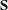as in Dempster, Laird, and Rubin (1977). Becauseis not observable, it is frequently referred to as a latent variable. The unobservable variableassigns an observation to a component in the mixture model. The number of states, k, might be unknown, but it is known to be finite. Conditioning on the latent variable, the component memberships of each observation is assumed to be known, and Bayesian estimation is straightforward for each component in the finite mixture model. That is, conditional on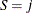, the distribution of the response is now assumed to be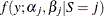. In other words, each distinct state of the random variableleads to a distinct set of parameters. The parameters in each component individually are then updated using a conjugate Gibbs sampler (where available) or a Metropolis-Hastings sampling algorithm.

The FMM procedure assumes that the random variablehas a discrete multinomial distribution with probability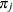of belonging to a component j; it can occupy one of k states. The distribution for the latent variableis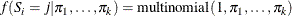where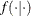denotes a conditional probability density. The parameters in the densitydenote the probability that S takes on state j.

The FMM procedure assumes a conjugate Dirichlet prior distribution on the mixture proportionswritten as: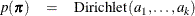where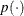indicates a prior distribution.

Using Bayes’ theorem, the likelihood function and prior distributions determine a conditionally conjugate posterior distribution ofand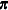from the multinomial distribution and Dirichlet distribution, respectively.